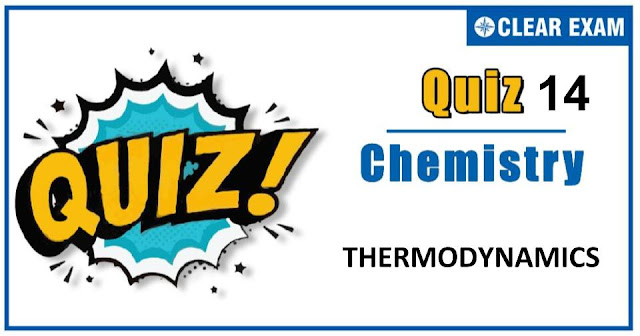## [LATEST]\$type=sticky\$show=home\$rm=0\$va=0\$count=4\$va=0

Thermodynamics is a very important branch of both chemistry and physics. It deals with the study of energy, the conversion of energy between different forms and the ability of energy to do work. Thermodynamics is an important chapter for JEE Mains, JEE Advance NEET and many others exams. As this chapter is present in both chemistry and physics and there is only a minor difference between them hence it becomes more important topic. It is not a tough topic, it only requires practice. This topic has been given good weightage in all engineering exams. So don't skip this and prepare well. All the best !.

Q1. For the reaction; C2H5OH(l) + 3O2(g) → 2CO2(g) + 3H2O(l), which one is true?
•  ΔH = ΔU − RT
•  ΔH = ΔU + RT
•  ΔH = ΔU + 2RT
•  ΔH = ΔU − 2RT
Solution:-
Δn = 2 − 3 = −1   ∴ ΔH = ΔU − RT

Q2. When two atoms of hydrogen combine to form a molecule of the hydrogen gas, the energy of the molecule is:
•  Greater than that of separate atoms
•  Equal to that of separate atoms
•  Lower than that of separate atoms
•  Sometimes lower and sometimes higher
Solution:-
Bond formation is always exothemic.

Q3. Energy equivalent to one erg, one joule and one calorie are in order:
•  1 erg > 1J > 1 cal
•  1 erg > 1 cal > 1J
•  1 cal > 1J > 1 erg
•  1J > 1 cal > 1 erg
Solution:-
1 cal = 4.185J = 4.18 × 107 erg

Q4. H2 + 1/2 O2 → H2O;   ΔH = −68.39 kcal.....(i)
K + aq. → KOH(aq) + 1/2H2;   ΔH = −48 kcal.....(ii)
KOH + aq. → KOH(aq);   ΔH = −14 kcal.....(iii)
The heat of formation (in kcal) of KOH is:
•  −68.39 + 48 −14
•  −68.39 − 48 + 14
•  68.39 − 48 + 14
•  68.39 + 48 + 14
Solution:-
K + 1/2O2 + 1/2H2 → KOH;   ΔH = ?
Find ΔH by eq.[(i)+(ii)]−(iii).

Q5. The standard heat of combustion of solid boron is:
•  Δf H°(B2O3)
•  1/2 Δf H°(B2O3)
•  −Δf H°(B2O3)
•  −1/2 Δf H°(B2O3)
Solution:-
2B + 3/2O2 → B2O3;   ΔfH°(B2O3)
∴ ΔcH°(B) = 1/2Δf H°(B2O3)
The heat is given out.

Q6. The ΔH°ionisation for HCN and CH3COOH are 45.2 and 2.1 kJ/mol. Which of the following is correct?
•  pKαHCN < pKαCH3COOH
•  pKαHCN > pKαCH3COOH
•  pKαHCN = pKαCH3COOH
•  None of the above
Solution:-
More is heat of ionization of acid, more is stability of acid or lesser is dissosciation or KαCH3COOH > KαHCN. Thus, pKαHCN > pKαCH3COOH

Q7. During an isothermal expansion of an ideal gas its:
•  Enthalpy decreases
•  Internal energy decreases
•  Internal energy increases
•  Internal energy remains constant
Solution:-
At isothermal condition, T = constant.

Q8. The heat of combustion for C, H2 and CH4 are −349.0, −241.8 and −906.7 kJ respectively. The heat of formation of CH4 is:
•  174.1 kJ
•  274.1 kJ
•  374.1 kJ
•  74.1 kJ
Solution:-
By eq.[(i) + 2×(ii)]−(iii),
C + 2H2 → CH4;   ΔH = 74.1kJ

Q9. The heat change for the reaction, C(s) + 2S(s) → CS2(l) is called
•  Heat of solution of CS2
•  Heat of fusion of CS2
•  Heat of formation of CS2
•  Heat of combustion of carbon
Solution:-
CS2 is formed from its initial components carbon and hydrogen.

Q10. When a solid melts, there is:
•  An increase in enthalpy
•  No change in enthalpy
•  A decrease in enthalpy
•  A decrease in internal energy
Solution:-
Ice takes up heat to melt and thus, enthalpy change is +ve.## Want to know more

Please fill in the details below:

## Latest NEET Articles\$type=three\$c=3\$author=hide\$comment=hide\$rm=hide\$date=hide\$snippet=hide

Name

ltr
item
BEST NEET COACHING CENTER | BEST IIT JEE COACHING INSTITUTE | BEST NEET & IIT JEE COACHING: Thermodynamics Quiz-14
Thermodynamics Quiz-14
https://1.bp.blogspot.com/-eFlgDTVXpNs/X5lzaCac53I/AAAAAAAAB4U/h72Da_smgR8F8tkbh3q0ZWq3HqaQYkZiwCLcBGAsYHQ/w640-h336/Quiz%2BImage%2B20%2B%252810%2529.jpg
https://1.bp.blogspot.com/-eFlgDTVXpNs/X5lzaCac53I/AAAAAAAAB4U/h72Da_smgR8F8tkbh3q0ZWq3HqaQYkZiwCLcBGAsYHQ/s72-w640-c-h336/Quiz%2BImage%2B20%2B%252810%2529.jpg
BEST NEET COACHING CENTER | BEST IIT JEE COACHING INSTITUTE | BEST NEET & IIT JEE COACHING
https://www.cleariitmedical.com/2020/10/thermodynamics-quiz-4.html
https://www.cleariitmedical.com/
https://www.cleariitmedical.com/
https://www.cleariitmedical.com/2020/10/thermodynamics-quiz-4.html
true
7783647550433378923
UTF-8

STAY CONNECTED